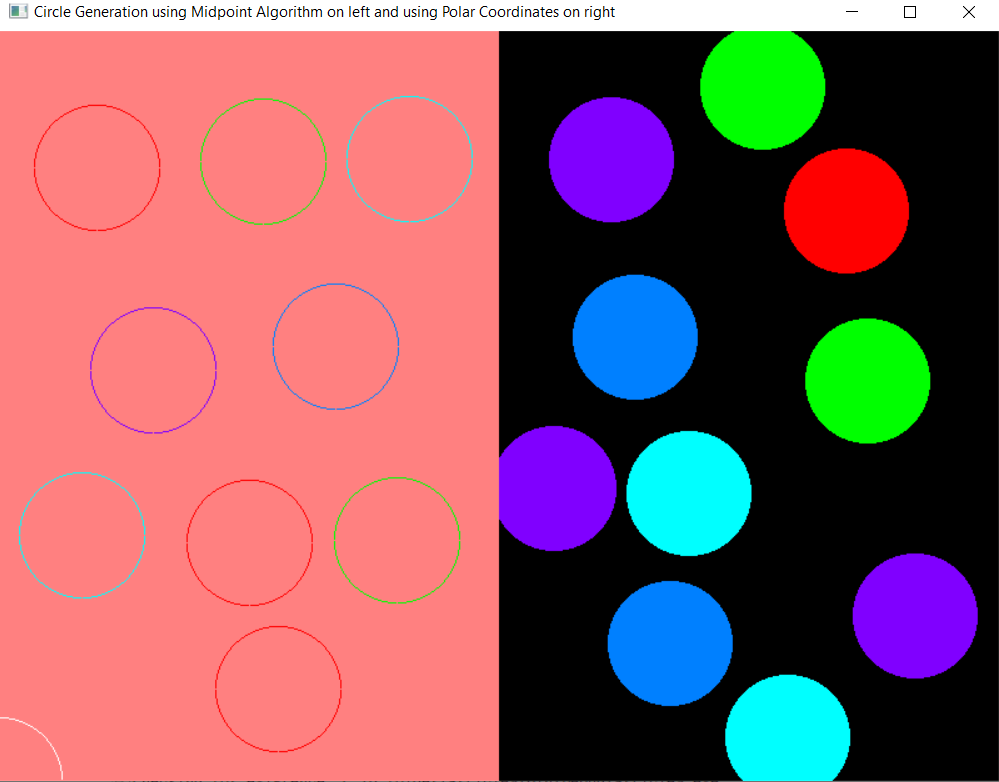Draw circles using the polar coordinates and mid-point circle drawing algorithm on the same console using openGL in C++

• Last Updated : 16 Jul, 2021

In this article, the task is to draw circles in two different subwindows using two different algorithms, and the circles are created using a single mouse click.

Approach:

• There are two subwindows and both of them use different algorithms to create circles.
• The left subwindow creates a circle using Midpoint Circle drawing algorithm and the right subwindow implements the concept of polar coordinates.
• A circle can be created anywhere on the console using a single left mouse click and the coordinates of the center of the circle created depends on the position of the click.
• To change the color of the circle, right-click on the mouse.
• After performing all operations, jump out of the program by simply pressing the Esc key on the keyboard.

Below is the implementation of the above approach:

C++

 // C++ program to implement onClick// functionality in OpenGL to draw// a circle using polar coordinates// and midpoint algorithm#include #include #include #include #define xpix 500#include using namespace std; float r, g, b, x, y;bool flag = true;int counter = 0; // Function to plot the coordinatesvoid plot(int x1, int y1){    // Initialize the points    glBegin(GL_POINTS);     // Draw the vertex    glVertex2i(x1 + x, y1 + y);     // End    glEnd();} // Function works on mouse clickvoid mouse(int button, int state,           int mousex, int mousey){    // Check if the current click    // is left-click or not    if (button == GLUT_LEFT_BUTTON        && state == GLUT_DOWN) {        flag = true;        x = mousex;        y = 600 - mousey;    }     // Check if the current click    // is right-click or not    else if (button == GLUT_RIGHT_BUTTON             && state == GLUT_DOWN) {        if (counter > 4) {            counter = 0;        }         counter++;         // Redisplay        glutPostRedisplay();    }} // Function that exits from programvoid keyboard(unsigned char key,              int x, int y){    switch (key) {    case 27:        glutHideWindow();    }} // Function to draw the circles using// the midpoint circle algorithmsvoid midPointCircleDraw(void){    // Change the color of circles on    // the right click    if (counter == 1) {        glColor3f(1, 0, 0);    }    else if (counter == 2) {        glColor3f(0, 1, 0);    }    else if (counter == 3) {        glColor3f(0, 1, 1);    }    else if (counter == 4) {        glColor3f(0.5, 0, 1);    }    else if (counter == 5) {         glColor3f(0, 0.5, 1);    }     // Matrix mode    glMatrixMode(GL_PROJECTION);    glLoadIdentity();     // Given the coordinates    gluOrtho2D(0.0, 400.0, 0.0, 600.0);    int r = 50;     if (flag) {         // Begin the pointer        glBegin(GL_POLYGON);        int x1 = 0;        int y1 = r;         // Initialising the value        // of the decision        float decision = 5 / 4 - r;         // Print the initial point on        // the axes after translation        plot(x1, y1);         while (y1 > x1) {             // Mid-point is inside or            // on the perimeter            if (decision < 0) {                x1++;                decision += 2 * x1 + 1;            }             // Mid-point is outside            // the perimeter            else {                y1--;                x1++;                decision += 2 * (x1 - y1) + 1;            }             // Plot the points in the            // different octants            plot(x1, y1);            plot(x1, -y1);            plot(-x1, y1);            plot(-x1, -y1);            plot(y1, x1);            plot(-y1, x1);            plot(y1, -x1);            plot(-y1, -x1);        }    }     glFlush();} // Function to draw the circle using// the polar coordinatesvoid polarCoordinateCircleDraw(void){    float angle_theta;     // Change the color of the circles    // on right click    if (counter == 1) {        glColor3f(1, 0, 0);    }    else if (counter == 2) {        glColor3f(0, 1, 0);    }    else if (counter == 3) {        glColor3f(0, 1, 1);    }    else if (counter == 4) {        glColor3f(0.5, 0, 1);    }    else if (counter == 5) {         glColor3f(0, 0.5, 1);    }     // Matrix mode    glMatrixMode(GL_PROJECTION);    glLoadIdentity();     // Given the coordinates    gluOrtho2D(0.0, 800.0, 0.0, 600.0);    if (flag) {         // Begin the pointer        glBegin(GL_POLYGON);         // Iterate through all the        // 360 degrees        for (int i = 0; i < 360; i++) {             angle_theta = i * 3.142 / 180;            glVertex2f(x + 50 * cos(angle_theta),                       y + 50 * sin(angle_theta));        }         // Set the vertex        glEnd();    }     // Flushes the frame buffer to    // the screen    glFlush();} // Driver Codeint main(int argc, char** argv){    // Initialize the drivers    glutInit(&argc, argv);     // Initialize the display mode    glutInitDisplayMode(        GLUT_SINGLE | GLUT_RGB);     // Update the window size    glutInitWindowSize(800, 600);     // Update the window position    glutInitWindowPosition(100, 100);     int mainWindow = glutCreateWindow(        "Circle Generation using "        "Midpoint Algorithm on "        "left and using Polar "        "Coordinates on right");     // Set the background color of    // the right sub-window    glClearColor(0, 0, 0, 0);     // Clears the frame buffer    glClear(GL_COLOR_BUFFER_BIT);     // Links display event with the    // display the event handler    // (polarCoordinateCircleDraw)    glutDisplayFunc(        polarCoordinateCircleDraw);     // Mouse event handler    glutMouseFunc(mouse);     // Create the sub-window to implement    // midpoint algorithm on left    int subWindow1        = glutCreateSubWindow(            mainWindow, 0, 0, 400, 600);     glutInitWindowPosition(100, 100);     // Sets the background color of    // the left sub-window    glClearColor(1, 0.5, 0.5, 1);     // Clears the frame buffer    glClear(GL_COLOR_BUFFER_BIT);     // Links display event with the    // display the event handler    // (midPointCircleDraw)    glutDisplayFunc(midPointCircleDraw);     // Mouse event handler    glutMouseFunc(mouse);     // Keyboard event handler    glutKeyboardFunc(keyboard);     // Loops the current event    glutMainLoop();}

Output:My Personal Notes arrow_drop_up